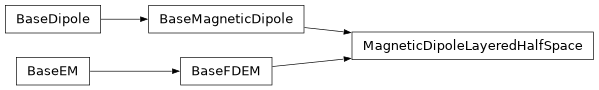# geoana.em.fdem.MagneticDipoleLayeredHalfSpace#class geoana.em.fdem.MagneticDipoleLayeredHalfSpace(frequency, thickness, **kwargs)#

Simulation class for a harmonic magnetic dipole over a layered halfspace.

This class is used to simulate the fields produced by a harmonic magnetic dipole source over a layered halfspace.

Parameters
thicknessNone or (‘*’) numpy.ndarray

Layer thicknesses (m) from the top layer downwards. If None is entered, you are defining a homogeneous halfspace. The bottom layer extends to infinity, thus n_layer = n_thickness + 1.

sigma(n_layer) np.ndarray or (n_layer, n_frequency) np.ndarray

Electrical conductivities for all layers (and at all frequencies). For non-dispersive conductivity (no chargeability) or for an instance of MagneticDipoleLayeredHalfSpace at a single frequency, sigma is assigned with a (n_layer) np.ndarray. For dispersive conductivity and multiple frequencies, sigma is assigned with a (n_layer, n_frequency) np.ndarray.

mu(n_layer) np.ndarray or (n_layer, n_frequency) np.ndarray

Magnetic permeability for all layers (and at all frequencies). For non-dispersive permeability (no viscous remanent magnetization) or for an instance of MagneticDipoleLayeredHalfSpace at a single frequency, mu is assigned with a (n_layer) np.ndarray. For dispersive permeability and multiple frequencies, mu is assigned with a (n_layer, n_frequency) np.ndarray.

epsilon(n_layer) np.ndarray or (n_layer, n_frequency) np.ndarray

Dielectric permittivity for all layers (and at all frequencies). Only applicable when quasistatic == True. For non-dispersive permittivity or for an instance of MagneticDipoleLayeredHalfSpace at a single frequency, epsilon is assigned with a (n_layer) np.ndarray. For dispersive permittivity and multiple frequencies, epsilon is assigned with a (n_layer, n_frequency) np.ndarray.

Attributes

 epsilon Dielectric permittivity for all layers (and frequencies) frequency Frequency (Hz) used for all computations location Location of the dipole moment Amplitude of the dipole moment of the magnetic dipole ($$A/m^2$$) mu Magnetic permeability for all layers (and frequencies) omega Angular frequency orientation Orientation of the dipole as a normalized vector sigma Electrical conductivity for all layers (and frequencies) sigma_hat Conductivity including electric displacement term. skin_depth Returns the skin depth for an electromagnetic wave in a homogeneous isotropic medium. thickness Thicknesses (m) for all layers from top to bottom wavenumber Wavenumber for an electromagnetic planewave in a homogenous isotropic medium.

Methods

 Cross products between a gridded set of vectors and the orientation of the source. distance(xyz) Scalar distance from dipole to a set of gridded xyz locations Dot product between the orientation of the source and a gridded set of vectors. magnetic_field(xyz[, field]) Compute the magnetic field produced by a magnetic dipole over a layered halfspace. Vector distance to a set of gridded xyz locations.